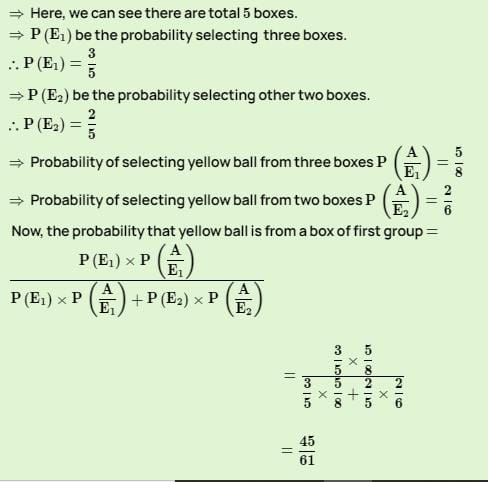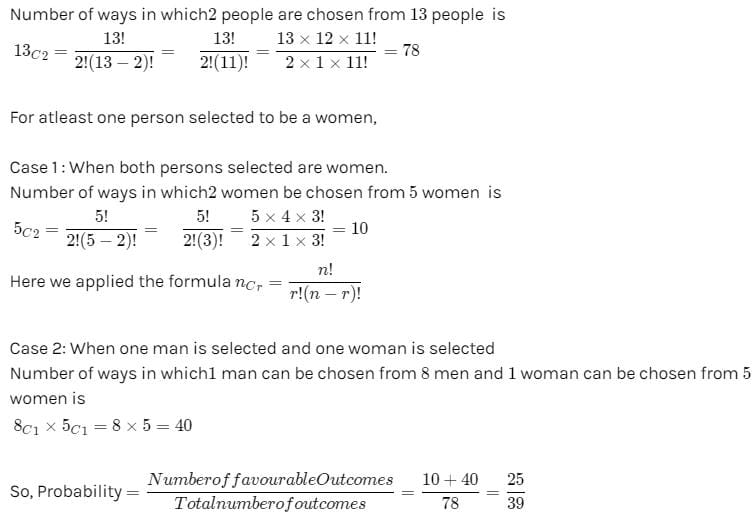Probability - MCQ 1

# Probability - MCQ 1

Test Description

## 20 Questions MCQ Test Quantitative Aptitude for Competitive Examinations | Probability - MCQ 1

Probability - MCQ 1 for Quant 2022 is part of Quantitative Aptitude for Competitive Examinations preparation. The Probability - MCQ 1 questions and answers have been prepared according to the Quant exam syllabus.The Probability - MCQ 1 MCQs are made for Quant 2022 Exam. Find important definitions, questions, notes, meanings, examples, exercises, MCQs and online tests for Probability - MCQ 1 below.
Solutions of Probability - MCQ 1 questions in English are available as part of our Quantitative Aptitude for Competitive Examinations for Quant & Probability - MCQ 1 solutions in Hindi for Quantitative Aptitude for Competitive Examinations course. Download more important topics, notes, lectures and mock test series for Quant Exam by signing up for free. Attempt Probability - MCQ 1 | 20 questions in 15 minutes | Mock test for Quant preparation | Free important questions MCQ to study Quantitative Aptitude for Competitive Examinations for Quant Exam | Download free PDF with solutions
 1 Crore+ students have signed up on EduRev. Have you?
Probability - MCQ 1 - Question 1

### A card from a pack of 52 cards is lost. From the remaining cards of the pack, two cards are drawn and are found to be both hearts. Find the Probability of the lost card being a heart?

Detailed Solution for Probability - MCQ 1 - Question 1

Total cards = 52
Drawn cards(Heart) = 2
Present total cards = total cards-drawn cards =52-2=50
Remaining Card 13-2 = 11
Probability = 11/50

Probability - MCQ 1 - Question 2

### There are three boxes each containing 3 Pink and 5 Yellow balls and also there are 2 boxes each containing 4 Pink and 2 Yellow balls. A Yellow ball is selected at random. Find the probability that Yellow ball is from a box of the first group?

Detailed Solution for Probability - MCQ 1 - Question 2Probability - MCQ 1 - Question 3

### A fruit basket contains 10 Guavas and 20 Bananas out of which 3 Guavas and 5 Bananas are defective. If two fruits selected at random, what is the probability that either both are Bananas or both are non-defective?

Detailed Solution for Probability - MCQ 1 - Question 3

P(A) = 20c2 / 30c2, P(B) = 22c2 / 30c2
P(A∩B) = 15c2 / 30c2
P(A∪B) = P(A) + P(B) – P(A∩B) ⇒ (20c2/30c2)+(22c2/30c2)- (15c2/30c2)=316/435

Probability - MCQ 1 - Question 4

A committee of five persons is to be chosen from a group of 10 people. The probability that a certain married couple will either serve together or not at all is?

Detailed Solution for Probability - MCQ 1 - Question 4

Five persons is to be chosen from a group of 10 people = 10C5 = 252
Couple Serve together = 8C3 * 2C2 = 56
Couple does not serve = 8C5 = 56
Probability = 102/252 = 51/126

Probability - MCQ 1 - Question 5

Out of 13 applicants for a job there are 5 women and 8 men. It is desired to select 2 persons for the job. The probability that at least one of the selected persons will be a woman is

Detailed Solution for Probability - MCQ 1 - Question 5Probability - MCQ 1 - Question 6

Three Bananas and three oranges are kept in a box. If two fruits are chosen at random, Find the probability that one is Banana and another one is orange?

Detailed Solution for Probability - MCQ 1 - Question 6

Total probability = 6C2 = 15
Probability that one is Banana and another one is orange = 3C1 * 3C1 = 9
probability = 9/15 = 3/5

Probability - MCQ 1 - Question 7

A basket contains 6 White 4 Black 2 Pink and 3 Green balls. If three balls picked up random, What is the probability that all three are White?

Detailed Solution for Probability - MCQ 1 - Question 7

Total Balls = 15
Probability = 6c3 / 15c3 = 4/91

Probability - MCQ 1 - Question 8

A basket contains 6 White 4 Black 2 Pink and 3 Green balls. If three balls are picked at random, what is the probability that two are Black and one is Green?

Detailed Solution for Probability - MCQ 1 - Question 8

Total Balls = 15
Probability = 4c2 * 3c1/ 15c3 = 18/455

Probability - MCQ 1 - Question 9

A basket contains 6 White 4 Black 2 Pink and 3 Green balls. If four balls are picked at random, what is the probability that atleast one is Black?

Detailed Solution for Probability - MCQ 1 - Question 9

Total Balls = 15
Probability = 11c4/15c4 = 22/91
One is black = 1 – 22/91 = 69/91

Probability - MCQ 1 - Question 10

A basket contains three blue and four red balls. If three balls are drawn at random from the basket, what is the probability that all the three balls are either blue or red?

Probability - MCQ 1 - Question 11

A box contains 27 marbles some are blue and others are green. If a marble is drawn at random from the box, the probability that it is blue is 1/3. Then how many number of green marbles in the box?

Detailed Solution for Probability - MCQ 1 - Question 11

Blue marble – x
xc1/27c1 = 1/3
x/27=1/3 → x=27/3=9
No of green marbles = Total – Blue marble =27-9=18

Probability - MCQ 1 - Question 12

In a bag there are 4 white, 4 red and 2 green balls. Two balls are drawn at random.What is the probability that at least one ball is of red colour?

Detailed Solution for Probability - MCQ 1 - Question 12

Total Balls =10
Other than red ball = 6c2
6c2/10c2=1/3 → 1-1/3 = 2/3

Probability - MCQ 1 - Question 13

Sahil has two bags (A & B) that contain green and blue balls.In the Bag ‘A’ there are 6 green and 8 blue balls and in the Bag ‘B’ there are 6 green and 6 blue balls. One ball is drawn out from any of these two bags. What is the probability that the ball drawn is blue?

Detailed Solution for Probability - MCQ 1 - Question 13

Total balls in A bag = 14, Total balls in A bag = 12
A bag = 1/2(8c1/14c1) = 2/7
B bag = 1/2(6c1/12c1) = 1/4 → total Probability = 2/7 + 1/4 =15/28

Probability - MCQ 1 - Question 14

In an examination, there are three sections namely Reasoning, Maths and English. Reasoning part contains 4 questions. There are 5 questions in maths section and 6 questions in English section. If three questions are selected randomly from the list of questions then what is the probability that all of them are from maths?

Detailed Solution for Probability - MCQ 1 - Question 14

Total no of questions= 15
Probability = 5c3/15c3 = 2/91

Probability - MCQ 1 - Question 15

A basket contains 5 red 4 blue 3 green marbles. If three marbles picked up random, What is the probability that either all are green or all are red?

Detailed Solution for Probability - MCQ 1 - Question 15

Total Marbles = 12
Either all are green or all are red = 5c3 + 3c3
probability = 5c3 + 3c3/12c3 = 11/220 = 1/20

Probability - MCQ 1 - Question 16

A basket contains 5 red 4 blue 3 green marbles. If three marbles picked up random, What is the probability that at least one is blue?

Detailed Solution for Probability - MCQ 1 - Question 16

Total Marbles = 12
other than blue 8c3 / 12c3 = 14/55
probability = 1-14/55 = 41/55

Probability - MCQ 1 - Question 17

A basket contains 5 red 4 blue 3 green marbles. If two marbles picked up random, What is the probability that both are red?

Detailed Solution for Probability - MCQ 1 - Question 17

Total Marbles = 12
Probability = 5c2 / 12c2 = 5/33

Probability - MCQ 1 - Question 18

A bag contains 5 red caps, 4 blue caps, 3 yellow caps and 2 green caps.If three caps are picked at random, what is the probability that two are red and one is green?

Detailed Solution for Probability - MCQ 1 - Question 18

Total caps = 14
Probability = 5c2 * 2c1/ 14c3 = 5/91

Probability - MCQ 1 - Question 19

A bag contains 5 red caps, 4 blue caps, 3 yellow caps and 2 green caps. If four caps are picked at random, what is the probability that two are red, one is blue and one is green?

Detailed Solution for Probability - MCQ 1 - Question 19

Total caps = 14
Probability = 5c2 * 4c1 * 2c1 / 14c4 = 80/1001

Probability - MCQ 1 - Question 20

A bag contains 2 red caps, 4 blue caps, 3 yellow caps and 5 green caps. If three caps are picked at random, what is the probability that none is green?

Detailed Solution for Probability - MCQ 1 - Question 20

Total caps = 14
Probability = 5c0 * 9c3/ 14c3 = 3/13

## Quantitative Aptitude for Competitive Examinations

33 videos|34 docs|140 tests
 Use Code STAYHOME200 and get INR 200 additional OFF Use Coupon Code
Information about Probability - MCQ 1 Page
In this test you can find the Exam questions for Probability - MCQ 1 solved & explained in the simplest way possible. Besides giving Questions and answers for Probability - MCQ 1, EduRev gives you an ample number of Online tests for practice

## Quantitative Aptitude for Competitive Examinations

33 videos|34 docs|140 tests